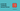# JS Challenge 3: Remove ZeroesIn this article we will solve together the Remove Zeroes challenge from CodeWars, you can find it at this link. The difficulty of this challenge is medium.

Write a function that takes an array of values and moves all elements that are zero to the end of the array, otherwise preserving the order of the array. The zero elements must also maintain the order in which they occurred. For example, the following array [7, 2, 3, 0, 4, 6, 0, 0, 13, 0, 78, 0, 0, 19, 14] is transformed into [7, 2, 3, 4, 6, 13, 78, 19, 14, 0, 0, 0, 0, 0, 0]

Zero elements are defined by either 0 or "0". Some tests may include elements that are not number literals. You are NOT allowed to use any temporary arrays or objects. You are also not allowed to use any Array.prototype or Object.prototype methods.

If it were not for the last point regarding temporary arrays this challenge would have been easier as we could have completed it like this:

``````function removeZeros(array) {
const tail = []
for (const e of array) {
if (e === 0 || e === "0") {
tail[tail.length] = e
} else {
}
}
}``````

This solution is not mine, I took it from the challenge page. Unfortunately, it is not valid as we are not allowed to define new arrays where to store the zeroes and the non-zeroes values.

In real life coding, where everything is valid, this is a perfectly fine solution so feel free to use it if you ever encounter a similar problem.

The challenge also forbids Array.prototype or Object.prototype methods so no push,slice,forEach etc..!

The way we are going to solve it without creating new arrays is simple, we are still going to iterate over the Array but instead of storing the values in temporary arrays, grab each zero and push it at the appropriate spot.

Let' start:

``````let limit = array.length;
let tmp;
for (let i = 0; i < limit; i++) {
if (array[i] === 0 || array[i] === "0") {

}
}``````

Now that we are iterating over the array what we need to do is to move the zero at the end but also move all the other values a step back too.

``````let limit = array.length;
let tmp;
for (let i = 0; i < limit; i++) {
if (array[i] === 0 || array[i] === "0") {
tmp = array[i];
// iterate again over the array
for (let j = i--; j < array.length-1; j++) {
array[j] = array[j+1];
}
}
}``````
JS Challenge 4: Who Likes it?

The new For Loop that we added is iterating again over the Array, moving items back one position, look at this example:

``````// before our loop
[1,2,0,3,4,5]
// after our loop
[1,2,3,4,5,5]``````

As you can see, our loop will move every value back one spot and we will then put the zero back at the end, replacing the now duplicate final value.

To save the zero value we created a `tmp` variable because we need to know if it's an integer 0 or a string '0'.

Let's finalize the function like so:

``````function removeZeros(array) {
let limit = array.length;
let tmp;
for (let i = 0; i < limit; i++) {
if (array[i] === 0 || array[i] === "0") {
tmp = array[i];
// iterate again over the array
for (let j = i--; j < array.length-1; j++) {
array[j] = array[j+1];
}
// replace last value with the zero
array[array.length-1] = tmp;
limit --;
}
}
return array;
}``````

After we moved everything back one place we replace the last value with `array[array.length-1] = tmp;`

If you are wondering why are we reducing the `limit` variable at the end of the loop it's because we are moving zeroes at the back of the array so we are effectively reducing the portion of the array that needs to be checked by one after each iteration where zero is found.

This is an example:

``````let array =  [1,2,'0',3,0,4,5];
// after one iteration where a zero is found
// [1,2,3,0,4,5,'0'];
// after another iteration where a zero is found
// [1,2,3,4,5,'0',0];
// if we don't reduce the size of the iteration we end up with one more iteration like this
// [1,2,3,4,5,0,'0'];``````

If you see in the above example, if we don't reduce the size of the iterable array, we will end up with one more iteration where we will push the '0' at the back, resulting in a wrong result as we are not respecting the correct order.

That is why we are calling `limit --`.

There are many other ways of solving this problem, let me know yours in the comment.

If you liked this type of content, please let me know in the comments and I'll create more of these.